Demo problem: Turek & Hron's FSI benchmark problem

In this example we consider the flow in a 2D channel past a cylinder with an attached elastic "flag". This is the FSI benchmark problem proposed by Turek & Hron,

"Proposal for Numerical Benchmarking of Fluid-Structure Interaction between an Elastic Object and a Laminar Incompressible Flow", S. Turek & J. Hron, pp. 371-385. In: "Fluid-Structure Interaction" Springer Lecture Notes in Computational Science and Engineering 53. Ed. H.-J. Bungartz & M. Schaefer. Springer Verlag 2006.

The problem combines the two single-physics problems of

This is our first example problem that involves the coupling between a fluid and "proper" solid (rather than beam structure) and also includes both fluid and wall inertia.

The problem presented here was used as one of the test cases for oomph-lib's FSI preconditioner; see

Heil, M., Hazel, A.L. & Boyle, J. (2008): Solvers for large-displacement fluid-structure interaction problems: Segregated vs. monolithic approaches. Computational Mechanics.

In this tutorial we concentrate on the problem formulation. The application of the preconditioner is discussed elsewhere – the required source code is contained in the driver code.

# The Problem

The figure below shows a sketch of the problem: A 2D channel of height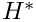and length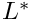conveys fluid of density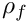and dynamic viscosity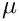and contains a cylinder of diameter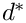, centred at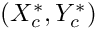to which a linearly elastic "flag" of thickness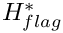and length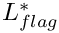is attached. Steady Poiseuille flow with average velocity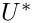is imposed at the left end of the channel while we assume the outflow to be parallel and axially traction-free. We model the flag as a linearly elastic Hookean solid with elastic modulus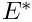, density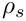and Poisson's ratio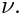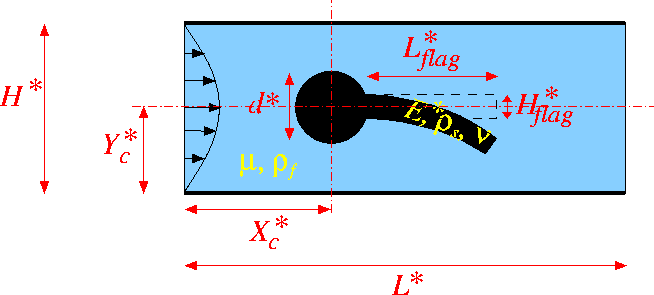Sketch of the problem in dimensional variables.

We non-dimensionalise all length and coordinates on the diameter of the cylinder,, the velocities on the mean velocity,, and the fluid pressure on the viscous scale. To facilitate comparisons with Turek & Hron's dimensional benchmark data (particularly for the period of the self-excited oscillations), we use a timescale of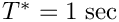to non-dimensionalise time. The fluid flow is then governed by the non-dimensional Navier-Stokes equations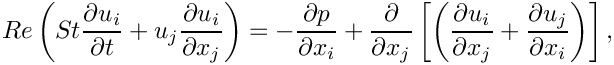where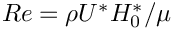and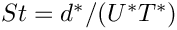, and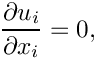subject to parabolic inflow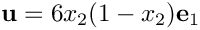at the inflow cross-section; parallel, axially-traction-free outflow at the outlet; and no-slip on the stationary channel walls and the surface of the cylinder,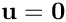. The no-slip condition on the moving flag is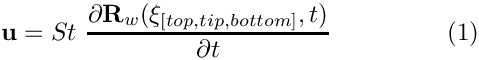where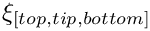are Lagrangian coordinates parametrising the three faces of the flag.

We describe the deformation of the elastic flag by the non-dimensional position vector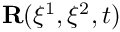which is determined by the principle of virtual displacements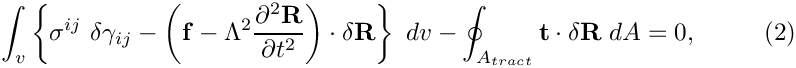where all solid stresses and tractions have been non-dimensionalised on Young's modulus,; see the Solid Mechanics Tutorial for details. The solid mechanics timescale ratio (the ratio of the timescale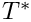chosen to non-dimensionalise time, to the intrinsic timescale of the solid) can be expressed in terms of the Reynolds and Strouhal numbers, the density ratio, and the FSI interaction parameter as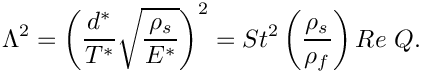Here is a sketch of the non-dimensional version of the problem: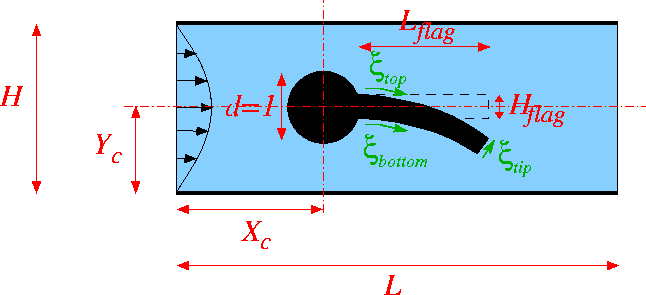Sketch of the fluid problem in dimensionless variables, showing the Lagrangian coordinates that parametrise the three faces of the flag.

# Parameter values for the benchmark problems

The (dimensional) parameter values given in Turek & Hron's benchmark correspond to the following non-dimensional parameters:

## Geometry

• Cylinder diameter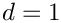• Centre of cylinder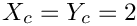• Channel length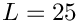• Channel width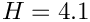• Thickness of the undeformed flag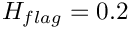• Right end of undeformed flag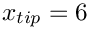## Non-dimensional parameters

The three FSI test cases correspond to the following non-dimensional parameters:

 ..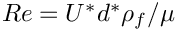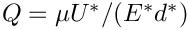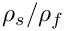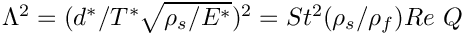FSI1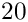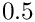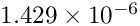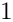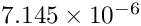FSI2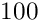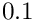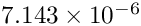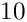FSI3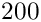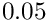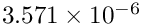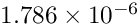# Results

The test cases FSI2 and FSI3 are the most interesting because the system develops large-amplitude self-excited oscillations

## FSI2

Following an initial transient period the system settles into large-amplitude self-excited oscillations during which the oscillating flag generates a regular vortex pattern that is advected along the channel. This is illustrated in the figure below which shows a snapshot of the flow field (pressure contours and instantaneous streamlines) at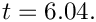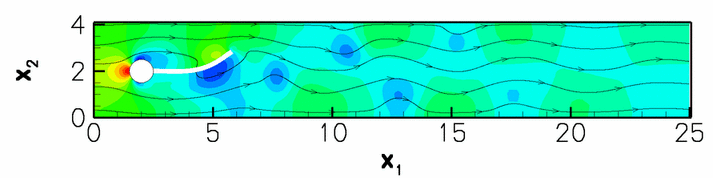Snapshot of the flow field (instantaneous streamlines and pressure contours)

The constantly adapted mesh contains and average of 65,000 degrees of freedom. A relatively large timestep of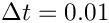– corresponding to about 50 timesteps per period of the oscillation – was used in this computation. With this discretisation the system settles into oscillations with a period of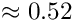and an amplitude of the tip-displacement of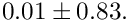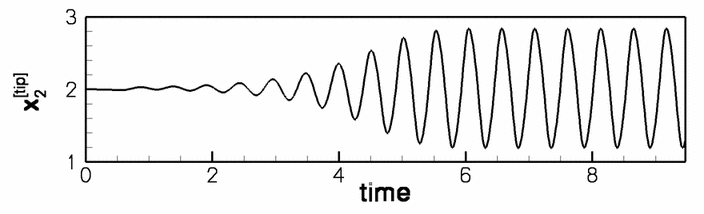Time trace of the tip displacement.

## FSI3

The figures below shows the corresponding results for the case FSI3 in which the fluid and solid densities are equal and the Reynolds number twice as large as in the FSI2 case. The system performs oscillations of much higher frequency and smaller amplitude. This is illustrated in the figure below which shows a snapshot of the flow field (pressure contours and instantaneous streamlines) at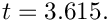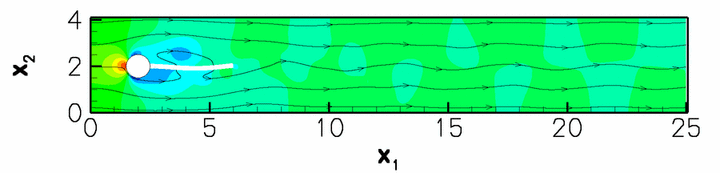Snapshot of the flow field (instantaneous streamlines and pressure contours)

This computation was performed with a timestep of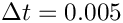and resulted in oscillations with a period of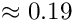and an amplitude of the tip-displacement of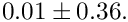The increase in frequency and Reynolds number leads to the development of thinner boundary and shear layers which require a finer spatial resolution, involving an average of 84,000 degrees of freedom.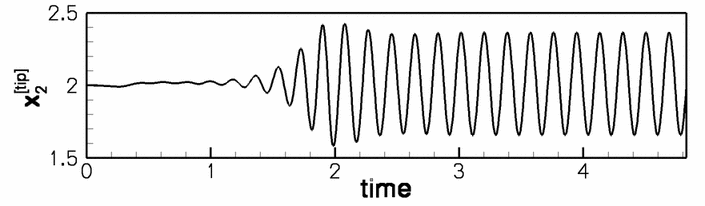Time trace of the tip displacement.

# Overview of the driver code

Since the driver code is somewhat lengthy we start by providing a brief overview of the main steps in the Problem construction:

1. We start by discretising the flag with 2D solid elements, as in the corresponding single-physics solid mechanics example.

2. Next we attach FSISolidTractionElements to the three solid mesh boundaries that are exposed to the fluid traction. These elements are used to compute and impose the fluid traction onto the solid elements, using the flow field from the adjacent fluid elements.

3. We now combine the three sets of FSISolidTractionElements into three individual (sub-)meshes and convert these to GeomObjects, using the MeshAsGeomObject class.

4. The GeomObject representation of the three surface meshes is then passed to the constructor of the fluid mesh. The algebraic node-update methodology provided in the AlgebraicMesh base class is used to update its nodal positions in response to the motion of its bounding GeomObjects.

5. Finally, we use the helper function FSI_functions::setup_fluid_load_info_for_solid_elements(...) to set up the fluid-structure interaction – this function determines which fluid elements are adjacent to the Gauss points in the FSISolidTractionElements that apply the fluid traction to the solid.

6. Done!

# Parameter values for the benchmark problems

As usual, We use a namespace to define the (many) global parameters, using default assignments for the FSI1 test case.

//=====start_of_global_parameters=================================
/// Global variables
//================================================================
{
/// Default case ID
string Case_ID="FSI1";
/// Reynolds number (default assignment for FSI1 test case)
double Re=20.0;
/// Strouhal number (default assignment for FSI1 test case)
double St=0.5;
/// Product of Reynolds and Strouhal numbers (default
/// assignment for FSI1 test case)
double ReSt=10.0;
/// FSI parameter (default assignment for FSI1 test case)
double Q=1.429e-6;
/// Density ratio (solid to fluid; default assignment for FSI1
/// test case)
double Density_ratio=1.0;
/// Height of flag
double H=0.2;
/// x position of centre of cylinder
double Centre_x=2.0;
/// y position of centre of cylinder
double Centre_y=2.0;
/// Pointer to constitutive law
ConstitutiveLaw* Constitutive_law_pt=0;
/// Timescale ratio for solid (dependent parameter
/// assigned in set_parameters())
double Lambda_sq=0.0;
/// Timestep
double Dt=0.1;
/// Ignore fluid (default assignment for FSI1 test case)
/// Elastic modulus
double E=1.0;
/// Poisson's ratio
double Nu=0.4;
Global variables.
Definition: turek_flag.cc:52
double Centre_x
x position of centre of cylinder
Definition: turek_flag.cc:78
Definition: turek_flag.cc:84
double Nu
Poisson's ratio.
Definition: turek_flag.cc:103
double Lambda_sq
Timescale ratio for solid (dependent parameter assigned in set_parameters())
Definition: turek_flag.cc:91
double Density_ratio
Density ratio (solid to fluid; default assignment for FSI1 test case)
Definition: turek_flag.cc:72
double Q
FSI parameter (default assignment for FSI1 test case)
Definition: turek_flag.cc:68
double ReSt
Product of Reynolds and Strouhal numbers (default assignment for FSI1 test case)
Definition: turek_flag.cc:65
string Case_ID
Default case ID.
Definition: turek_flag.cc:55
double Re
Reynolds number (default assignment for FSI1 test case)
Definition: turek_flag.cc:58
double E
Elastic modulus.
Definition: turek_flag.cc:100
Ignore fluid (default assignment for FSI1 test case)
Definition: turek_flag.cc:97
double Dt
Timestep.
Definition: turek_flag.cc:94
double H
Height of flag.
Definition: turek_flag.cc:75
ConstitutiveLaw * Constitutive_law_pt
Pointer to constitutive law.
Definition: turek_flag.cc:87
double St
Strouhal number (default assignment for FSI1 test case)
Definition: turek_flag.cc:61
double Centre_y
y position of centre of cylinder
Definition: turek_flag.cc:81

We also include a gravitational body force for the solid. (This is only used for the solid mechanics test cases, CSM1 and CSM2, which will not be discussed here.)

/// Non-dim gravity (default assignment for FSI1 test case)
double Gravity=0.0;
/// Non-dimensional gravity as body force
void gravity(const double& time,
const Vector<double> &xi,
Vector<double> &b)
{
b=0.0;
b=-Gravity;
}
void gravity(const double &time, const Vector< double > &xi, Vector< double > &b)
Non-dimensional gravity as body force.
Definition: turek_flag.cc:109
double Gravity
Non-dim gravity (default assignment for FSI1 test case)
Definition: turek_flag.cc:106

The domain geometry and flow field are fairly complex and it is difficult to construct a good initial guess for the Newton iteration. To ensure its convergence at the beginning of the simulation we therefore employ the method suggested by Turek & Hron: We start the flow from rest and ramp up the inflow profile from zero to its maximum value. The parameters for the time-dependent increase in the influx are defined here:

/// Period for ramping up in flux
double Ramp_period=2.0;
/// Min. flux
double Min_flux=0.0;
/// Max. flux
double Max_flux=1.0;
/// Flux increases between Min_flux and Max_flux over
/// period Ramp_period
double flux(const double& t)
{
if (t<Ramp_period)
{
0.5*(1.0-cos(MathematicalConstants::Pi*t/Ramp_period));
}
else
{
return Max_flux;
}
} // end of specification of ramped influx
double Max_flux
Max. flux.
Definition: turek_flag.cc:124
double flux(const double &t)
Flux increases between Min_flux and Max_flux over period Ramp_period.
Definition: turek_flag.cc:128
double Min_flux
Min. flux.
Definition: turek_flag.cc:121
double Ramp_period
Period for ramping up in flux.
Definition: turek_flag.cc:118

Finally, we provide a helper function that assigns the parameters for the various test cases, depending on their ID ("FSI1", "FSI2", "FSI3", "CSM1" or "CSM2"). Here is the assignment for the case FSI1:

/// Set parameters for the various test cases
void set_parameters(const string& case_id)
{
// Remember which case we're dealing with
Case_ID=case_id;
// Setup independent parameters depending on test case
if (case_id=="FSI1")
{
// Reynolds number based on diameter of cylinder
Re=20.0;
// Strouhal number based on timescale of one second
St=0.5;
// Womersley number
// FSI parameter
Q=1.429e-6;
// Timestep -- aiming for about 40 steps per period
Dt=0.1;
// Density ratio
// Gravity
Gravity=0.0;
// Max. flux
Max_flux=1.0;
// Ignore fluid
// Compute dependent parameters
// Timescale ratio for solid
}
void set_parameters(const string &case_id)
Set parameters for the various test cases.
Definition: turek_flag.cc:143

In the interest of brevity we omit the listings of the assignments for the other cases. Finally, we select the length of the time interval over which the influx is ramped up from zero to its maximum value to be equal to 20 timesteps, create a constitutive equation for the solid, and document the parameter values used in the simulation:

// Ramp period (20 timesteps)
// "Big G" Linear constitutive equations:
Constitutive_law_pt = new GeneralisedHookean(&Nu,&E);
// Doc
oomph_info << std::endl;
oomph_info << "-------------------------------------------"
<< std::endl;
oomph_info << "Case: " << case_id << std::endl;
oomph_info << "Re = " << Re << std::endl;
oomph_info << "St = " << St << std::endl;
oomph_info << "ReSt = " << ReSt << std::endl;
oomph_info << "Q = " << Q << std::endl;
oomph_info << "Dt = " << Dt << std::endl;
oomph_info << "Ramp_period = " << Ramp_period << std::endl;
oomph_info << "Max_flux = " << Max_flux << std::endl;
oomph_info << "Density_ratio = " << Density_ratio << std::endl;
oomph_info << "Lambda_sq = " << Lambda_sq << std::endl;
oomph_info << "Gravity = " << Gravity << std::endl;
oomph_info << "-------------------------------------------"
<< std::endl << std::endl;
}
}// end_of_namespace

# The driver code

The driver code has the usual structure, though in this case we use the command line arguments to indicate which case (FSI1, FSI2, FSI3, CSM1 or CSM2) to run. The absence of a command line argument is interpreted as the code being run as part of oomph-lib's self-test procedure in which case we perform a computation with the parameter values for case FSI1 and perform only a few timesteps.

//=======start_of_main==================================================
/// Driver
//======================================================================
int main(int argc, char* argv[])
{
// Store command line arguments
CommandLineArgs::setup(argc,argv);
// Get case id as string
string case_id="FSI1";
if (CommandLineArgs::Argc==1)
{
oomph_info << "No command line arguments; running self-test FSI1"
<< std::endl;
}
else if (CommandLineArgs::Argc==2)
{
case_id=CommandLineArgs::Argv;
}
else
{
oomph_info << "Wrong number of command line arguments" << std::endl;
oomph_info << "Enter none (for default) or one (namely the case id"
<< std::endl;
oomph_info << "which should be one of: FSI1, FSI2, FSI3, CSM1"
<< std::endl;
}
std::cout << "Running case " << case_id << std::endl;
int main(int argc, char *argv[])
Driver.
Definition: turek_flag.cc:1080

We set up the global parameter values, create a DocInfo object and trace file to record the output, and build the problem.

// Setup parameters for case identified by command line
// argument
// Prepare output
DocInfo doc_info;
ofstream trace_file;
doc_info.set_directory("RESLT");
trace_file.open("RESLT/trace.dat");
// Length and height of domain
double length=25.0;
double height=4.1;
//Set up the problem
RefineableQPVDElement<2,3> > problem(length, height);
//////////////////////////////////////////////////////////////////// ////////////////////////////////...
Definition: turek_flag.cc:371

Next, we choose the number of timesteps (using a smaller number for a validation run, and for the case FSI1 in which the system rapidly approaches a steady state) and initialise the time-stepping for an impulsive start from the zero flow solution.

// Default number of timesteps
unsigned nstep=4000;
{
std::cout << "Reducing number of steps for FSI1 " << std::endl;
nstep=400;
}
if (CommandLineArgs::Argc==1)
{
std::cout << "Reducing number of steps for validation " << std::endl;
nstep=2;
}
//Timestep:
// Initialise timestep
problem.initialise_dt(dt);
// Impulsive start
problem.assign_initial_values_impulsive(dt);

Finally, we document the initial condition and start the time-stepping procedure, setting the first flag to false because we have not specified an analytical expression for the initial conditions that could be re-assigned after the mesh adaptation when computing the first timestep.

// Doc the initial condition
problem.doc_solution(doc_info,trace_file);
doc_info.number()++;
// Don't re-set the initial conditions when adapting during first
// timestep
bool first = false;
// Max number of adaptation for time-stepping
for(unsigned i=0;i<nstep;i++)
{
// Solve the problem
// Output the solution
problem.doc_solution(doc_info,trace_file);
// Step number
doc_info.number()++;
}
trace_file.close();
}//end of main

# The Problem class

The Problem class contains the usual member functions, such as access functions to the various meshes. Because the nodal positions are updated by an algebraic node-update procedure, the function actions_before_newton_convergence_check() is employed to update the nodal positions in response to changes in the (solid) variables during the Newton iteration. The function actions_before_implicit_timestep() is used to adjust the influx during the start-up period.

//====start_of_problem_class===========================================
/// Problem class
//======================================================================
template< class FLUID_ELEMENT,class SOLID_ELEMENT >
class TurekProblem : public Problem
{
public:
/// Constructor: Pass length and height of domain
TurekProblem(const double &length, const double &height);
/// Access function for the fluid mesh
RefineableAlgebraicCylinderWithFlagMesh<FLUID_ELEMENT>* fluid_mesh_pt()
{ return Fluid_mesh_pt;}
/// Access function for the solid mesh
{return Solid_mesh_pt;}
/// Access function for the i-th mesh of FSI traction elements
SolidMesh*& traction_mesh_pt(const unsigned& i)
{return Traction_mesh_pt[i];}
/// Actions after adapt: Re-setup the fsi lookup scheme
/// Doc the solution
void doc_solution(DocInfo& doc_info, ofstream& trace_file);
/// Update function (empty)
/// Update function (empty)
/// Update the (dependent) fluid node positions following the
/// update of the solid variables before performing Newton convergence
/// check
/// Update the time-dependent influx
private:
/// Create FSI traction elements
/// Pointer to solid mesh
/// Pointer to fluid mesh
RefineableAlgebraicCylinderWithFlagMesh<FLUID_ELEMENT>* Fluid_mesh_pt;
/// Vector of pointers to mesh of FSI traction elements
Vector<SolidMesh*> Traction_mesh_pt;
/// Combined mesh of traction elements -- only used for documentation
/// Overall height of domain
double Domain_height;
/// Overall length of domain
double Domain_length;
/// Pointer to solid control node
/// Pointer to fluid control node
};// end_of_problem_class
Vector< SolidMesh * > Traction_mesh_pt
Vector of pointers to mesh of FSI traction elements.
Definition: turek_flag.cc:422
RefineableAlgebraicCylinderWithFlagMesh< FLUID_ELEMENT > * Fluid_mesh_pt
Pointer to fluid mesh.
Definition: turek_flag.cc:419
Pointer to solid mesh.
Definition: turek_flag.cc:416
double Domain_height
Overall height of domain.
Definition: turek_flag.cc:428
void doc_solution(DocInfo &doc_info, ofstream &trace_file)
Doc the solution.
Definition: turek_flag.cc:1008
Actions after adapt: Re-setup the fsi lookup scheme.
Definition: turek_flag.cc:895
RefineableAlgebraicCylinderWithFlagMesh< FLUID_ELEMENT > * fluid_mesh_pt()
Access function for the fluid mesh.
Definition: turek_flag.cc:379
Node * Fluid_control_node_pt
Pointer to fluid control node.
Definition: turek_flag.cc:437
void actions_before_implicit_timestep()
Update the time-dependent influx.
Definition: turek_flag.cc:869
TurekProblem(const double &length, const double &height)
Constructor: Pass length and height of domain.
Definition: turek_flag.cc:447
Access function for the solid mesh.
Definition: turek_flag.cc:383
SolidMesh *& traction_mesh_pt(const unsigned &i)
Access function for the i-th mesh of FSI traction elements.
Definition: turek_flag.cc:387
void actions_before_newton_solve()
Update function (empty)
Definition: turek_flag.cc:400
void actions_before_newton_convergence_check()
Update the (dependent) fluid node positions following the update of the solid variables before perfor...
Definition: turek_flag.cc:857
SolidMesh * Combined_traction_mesh_pt
Combined mesh of traction elements – only used for documentation.
Definition: turek_flag.cc:425
void create_fsi_traction_elements()
Create FSI traction elements.
Definition: turek_flag.cc:953
Node * Solid_control_node_pt
Pointer to solid control node.
Definition: turek_flag.cc:434
void actions_after_newton_solve()
Update function (empty)
Definition: turek_flag.cc:397
double Domain_length
Overall length of domain.
Definition: turek_flag.cc:431

# The problem constructor

We start by building the solid mesh, using an initial discretisation with 20 x 2 elements in the x- and y-directions. (The length of the flag is determined such that it emanates from its intersection with the cylinder and ends at x=6; The origin vector shifts the "lower left" vertex of the solid mesh so that its centreline is aligned with the cylinder.)

//=====start_of_constructor=============================================
/// Constructor: Pass length and height of domain
//======================================================================
template< class FLUID_ELEMENT,class SOLID_ELEMENT >
TurekProblem(const double &length,
const double &height) : Domain_height(height),
Domain_length(length)
{
// Increase max. number of iterations in Newton solver to
// accomodate possible poor initial guesses
Max_newton_iterations=20;
Max_residuals=1.0e4;
// Build solid mesh
//------------------
// # of elements in x-direction
unsigned n_x=20;
// # of elements in y-direction
unsigned n_y=2;
// Domain length in y-direction
// Create the flag timestepper (consistent with BDF<2> for fluid)
Newmark<2>* flag_time_stepper_pt=new Newmark<2>;
/// Left point on centreline of flag so that the top and bottom
/// vertices merge with the cylinder.
Vector<double> origin(2);
origin=Global_Parameters::Centre_y-0.5*l_y;
// Set length of flag so that endpoint actually stretches all the
// way to x=6:
double l_x=6.0-origin;
//Now create the mesh
n_x,n_y,l_x,l_y,origin,flag_time_stepper_pt);

We create an error estimator for the solid mesh and identify a control node at the tip of the flag to track its motion.

// Set error estimator for the solid mesh
Z2ErrorEstimator* solid_error_estimator_pt=new Z2ErrorEstimator;
solid_mesh_pt()->spatial_error_estimator_pt()=solid_error_estimator_pt;
// Element that contains the control point
FiniteElement* el_pt=solid_mesh_pt()->finite_element_pt(n_x*n_y/2-1);
// How many nodes does it have?
unsigned nnod=el_pt->nnode();
// Get the control node
Solid_control_node_pt=el_pt->node_pt(nnod-1);
std::cout << "Coordinates of solid control point "
<< Solid_control_node_pt->x(0) << " "
<< Solid_control_node_pt->x(1) << " " << std::endl;

Finally, we perform one uniform mesh refinement and disable any further mesh adaptation.

// Refine the mesh uniformly
solid_mesh_pt()->refine_uniformly();
//Do not allow the solid mesh to be refined again

Next, we attach FSISolidTractionElements to the boundaries of the solid mesh that are exposed to the fluid. We complete their build by specifying which boundary of the bulk mesh they are attached to, as this information is required when setting up the fluid-structure interaction; see Further comments and exercises.

// Build mesh of solid traction elements that apply the fluid
//------------------------------------------------------------
// traction to the solid elements
//-------------------------------
// Create storage for Meshes of FSI traction elements at the bottom
// top and left boundaries of the flag
Traction_mesh_pt.resize(3);
// Now construct the traction element meshes
Traction_mesh_pt=new SolidMesh;
Traction_mesh_pt=new SolidMesh;
Traction_mesh_pt=new SolidMesh;
// Build the FSI traction elements
create_fsi_traction_elements();
// Loop over traction elements, pass the FSI parameter and tell them
// the boundary number in the bulk solid mesh -- this is required so
for (unsigned bound=0;bound<3;bound++)
{
unsigned n_face_element = Traction_mesh_pt[bound]->nelement();
for(unsigned e=0;e<n_face_element;e++)
{
//Cast the element pointer and specify boundary number
FSISolidTractionElement<SOLID_ELEMENT,2>* elem_pt=
dynamic_cast<FSISolidTractionElement<SOLID_ELEMENT,2>*>
(Traction_mesh_pt[bound]->element_pt(e));
// Specify boundary number
elem_pt->set_boundary_number_in_bulk_mesh(bound);
// Function that specifies the load ratios
elem_pt->q_pt() = &Global_Parameters::Q;
}
} // build of FSISolidTractionElements is complete

Finally, we create GeomObject representations of the three surface meshes of FSISolidTractionElements. We will use these to represent the curvilinear, moving boundaries of the fluid mesh.

// Turn the three meshes of FSI traction elements into compound
// geometric objects (one Lagrangian, two Eulerian coordinates)
// that determine the boundary of the fluid mesh
MeshAsGeomObject*
bottom_flag_pt=
new MeshAsGeomObject
(Traction_mesh_pt);
MeshAsGeomObject* tip_flag_pt=
new MeshAsGeomObject
(Traction_mesh_pt);
MeshAsGeomObject* top_flag_pt=
new MeshAsGeomObject
(Traction_mesh_pt);

The final mesh to be built is the fluid mesh whose constructor requires pointers to the four GeomObjects that represent the cylinder and three fluid-loaded faces of the flag, respectively. We represent the cylinder by a Circle object:

// Build fluid mesh
//-----------------
//Create a new Circle object as the central cylinder
Circle* cylinder_pt = new Circle(Global_Parameters::Centre_x,

We build the mesh and identify a control node (a node at the upstream face of the cylinder), before creating an error estimator and performing one uniform mesh refinement.

// Allocate the fluid timestepper
BDF<2>* fluid_time_stepper_pt=new BDF<2>;
// Build fluid mesh
Fluid_mesh_pt=
new RefineableAlgebraicCylinderWithFlagMesh<FLUID_ELEMENT>
(cylinder_pt,
top_flag_pt,
bottom_flag_pt,
tip_flag_pt,
length, height,
fluid_time_stepper_pt);
// I happen to have found out by inspection that
// node 5 in the hand-coded fluid mesh is at the
// upstream tip of the cylinder
Fluid_control_node_pt=Fluid_mesh_pt->node_pt(5);
// Set error estimator for the fluid mesh
Z2ErrorEstimator* fluid_error_estimator_pt=new Z2ErrorEstimator;
fluid_mesh_pt()->spatial_error_estimator_pt()=fluid_error_estimator_pt;
// Refine uniformly
Fluid_mesh_pt->refine_uniformly();

We now add the various meshes to the Problem's collection of sub-meshes and combine them to a global mesh

// Build combined global mesh
//---------------------------
// Add Solid mesh the problem's collection of submeshes
for (unsigned i=0;i<3;i++)
{
}
// Build combined "global" mesh
build_global_mesh();

The application of boundary conditions for the solid are straightforward: All displacements of the flag's left end (mesh boundary 3) are suppressed; the other faces are free. Strictly speaking, the pinning of the redundant solid pressure nodes is superfluous since the RefineableQPVDElement used for the discretisation of the flag employ a displacement-based formulation, but it is good practise to perform this step anyway to "future-proof" the code for the use of other element types.

// Apply solid boundary conditons
//-------------------------------
//Solid mesh: Pin the left boundary (boundary 3) in both directions
unsigned n_side = mesh_pt()->nboundary_node(3);
//Loop over the nodes
for(unsigned i=0;i<n_side;i++)
{
solid_mesh_pt()->boundary_node_pt(3,i)->pin_position(0);
solid_mesh_pt()->boundary_node_pt(3,i)->pin_position(1);
}
// Pin the redundant solid pressures (if any)
PVDEquationsBase<2>::pin_redundant_nodal_solid_pressures(
solid_mesh_pt()->element_pt());

The fluid has Dirichlet boundary conditions (prescribed velocity) everywhere apart from the outflow where only the horizontal velocity is unknown.

// Apply fluid boundary conditions
//--------------------------------
//Fluid mesh: Horizontal, traction-free outflow; pinned elsewhere
unsigned num_bound = fluid_mesh_pt()->nboundary();
for(unsigned ibound=0;ibound<num_bound;ibound++)
{
unsigned num_nod= fluid_mesh_pt()->nboundary_node(ibound);
for (unsigned inod=0;inod<num_nod;inod++)
{
// Parallel, axially traction free outflow at downstream end
if (ibound != 1)
{
fluid_mesh_pt()->boundary_node_pt(ibound,inod)->pin(0);
fluid_mesh_pt()->boundary_node_pt(ibound,inod)->pin(1);
}
else
{
fluid_mesh_pt()->boundary_node_pt(ibound,inod)->pin(1);
}
}
}//end_of_pin
// Pin redundant pressure dofs in fluid mesh
RefineableNavierStokesEquations<2>::
pin_redundant_nodal_pressures(fluid_mesh_pt()->element_pt());

We impose a parabolic inflow profile with the current value of the influx at the inlet (fluid mesh boundary 3).

// Apply boundary conditions for fluid
//-------------------------------------
// Impose parabolic flow along boundary 3
// Current flow rate
double t=0.0;
double ampl=Global_Parameters::flux(t);
unsigned ibound=3;
unsigned num_nod= Fluid_mesh_pt->nboundary_node(ibound);
for (unsigned inod=0;inod<num_nod;inod++)
{
double ycoord = Fluid_mesh_pt->boundary_node_pt(ibound,inod)->x(1);
double uy = ampl*6.0*ycoord/Domain_height*(1.0-ycoord/Domain_height);
Fluid_mesh_pt->boundary_node_pt(ibound,inod)->set_value(0,uy);
Fluid_mesh_pt->boundary_node_pt(ibound,inod)->set_value(1,0.0);
}

We complete the build of the solid elements by passing them the pointer to the constitutive equation, the gravity vector and the timescale ratio:

// Complete build of solid elements
//---------------------------------
//Pass problem parameters to solid elements
unsigned n_element =solid_mesh_pt()->nelement();
for(unsigned i=0;i<n_element;i++)
{
//Cast to a solid element
SOLID_ELEMENT *el_pt = dynamic_cast<SOLID_ELEMENT*>(
solid_mesh_pt()->element_pt(i));
// Set the constitutive law
el_pt->constitutive_law_pt() =
//Set the body force
el_pt->body_force_fct_pt() = Global_Parameters::gravity;
// Timescale ratio for solid
el_pt->lambda_sq_pt() = &Global_Parameters::Lambda_sq;
}

The fluid elements require pointers to the Reynolds and Womersley (product of Reynolds and Strouhal) numbers:

// Complete build of fluid elements
//---------------------------------
// Set physical parameters in the fluid mesh
unsigned nelem=fluid_mesh_pt()->nelement();
for (unsigned e=0;e<nelem;e++)
{
// Upcast from GeneralisedElement to the present element
FLUID_ELEMENT* el_pt = dynamic_cast<FLUID_ELEMENT*>
(fluid_mesh_pt()->element_pt(e));
//Set the Reynolds number
el_pt->re_pt() = &Global_Parameters::Re;
//Set the Womersley number
el_pt->re_st_pt() = &Global_Parameters::ReSt;
}//end_of_loop